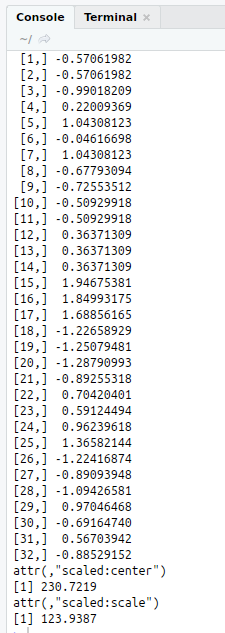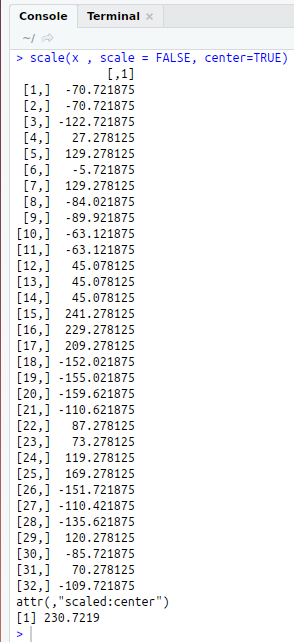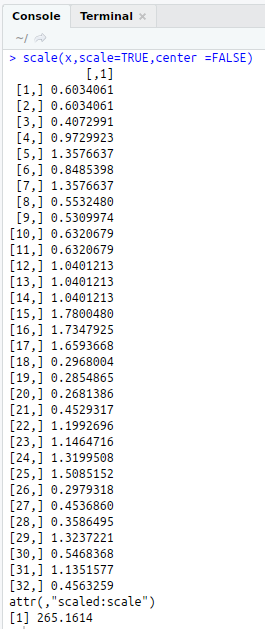• #5, First Floor, 4th Street Dr. Subbarayan Nagar Kodambakkam, Chennai-600 024 Landmark : Samiyar Madam
• pro@slogix.in
• +91- 81240 01111

#### Social List

##### How to implement Center and Scaling Function from caret Package in R?
###### Description

To implement the center and scaling function from caret package using R programming.

###### Process

Centering and scaling will work forcontinuous data.

#### Scale :

To scale the data, each predictor valueisdivided by its standard deviation(sd).

This helps in coercing the predictor valueto have a sdof one.

#### Center:

To center a predictor variable,the averagepredictor value is subtractedfrom allthe values.

As a result of centering, the predictorhas zero mean.

#### Package and Function:

R Package : caret

R Function : scale(x, scale =, center = )

x -- a Numeric Vector

scale -- logical value

center -- logical value

###### Sapmle Code

#Centering and Scaling

#install.packages(“caret”)
library(“caret”)
#Input
x<-mtcars\$disp

#Scale Value
scale(x,scale=TRUE,center =FALSE)

#Center Value
scale(x , scale = FALSE, center= TRUE)

#Both Scale and Center Value using Scale Function
scale(x, scale = TRUE, center = TRUE)

###### Screenshots